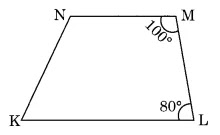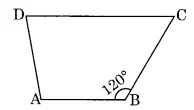NCERT Solutions for Class 8 Maths Chapter 3 Understanding Quadrilaterals Ex 3.3

# NCERT Solutions for Class 8 Maths Chapter 3 Understanding Quadrilaterals Ex 3.3

## NCERT Solutions for Class 8 Maths Chapter 3 Understanding Quadrilaterals Ex 3.3

NCERT Solutions for Class 8 Maths Chapter 3 Understanding Quadrilaterals Ex 3.3 are the part of NCERT Solutions for Class 8 Maths. Here you can find the NCERT Solutions for Class 8 Maths Chapter 3 Understanding Quadrilaterals Ex 3.3.

### Ex 3.3 Class 8 Maths Question 1.

Given a parallelogram ABCD. Complete each statement along with the definition or property used.
(ii)
DCB = ………
(iii) OC = ………
(iv) m
DAB + m CDA = ……..

Solution:

(i) AD = BC                [Opposite sides of a parallelogram are equal]
(ii)
DCB = BAD    [Opposite angles of a parallelogram are equal]
(iii) OC = OA             [Diagonals of a parallelogram bisect each other]
(iv) m
DAB + m CDA = 180°

[Adjacent angles of a parallelogram are supplementary]

### Ex 3.3 Class 8 Maths Question 2.

Consider the following parallelograms. Find the values of the unknowns x, y, z.

B = D      [Opposite angles of a parallelogram are equal]
D = 100°
y = 100°
A + B = 180°    [Adjacent angles of a parallelogram are supplementary]
z + 100° = 180°
z = 180° – 100° = 80°
A = C         [Opposite angles of a parallelogram are equal]
P + S = 180°            [Adjacent angles of parallelogram are supplementary]
x + 50° = 180°
x = 180° – 50° = 130°
Now,
P = R         [Opposite angles of a parallelogram are equal]
x = y
y = 130°
Also, y = z             [Alternate angles]
z = 130°
Hence, x = 130°, y = 130° and z = 130°
(iii) ABCD is a rhombus.
x = 90°        [ Diagonals bisect each other at 90°]
Now, in ∆OCB,
x + y + 30° = 180°     (Angle sum property)
90° + y + 30° = 180°
y + 120° = 180°
y = 180° – 120° = 60°
y = z                 (Alternate angles)
z = 60°
A + B = 180°    (Adjacent angles of a parallelogram are supplementary)
x + 80° = 180°
x = 180° – 80° = 100°
Now,
D = B        [Opposite angles of a parallelogram are equal]
y = 80°
Also, z =
B = 80°   (Corresponding angles)
D = B         [Opposite angles of a parallelogram are equal]
y = 112°
x + y + 40° = 180°         [Angle sum property]
x + 112° + 40° = 180°
x + 152° = 180°
x = 180° – 152 = 28°
z = x = 28°            (Alternate angles)
Hence x = 28°, y = 112°, z = 28°.

### Ex 3.3 Class 8 Maths Question 3.

Can a quadrilateral ABCD be a parallelogram if
(i)
D + B = 180°?
(ii) AB = DC = 8 cm, AD = 4 cm and BC = 4.4 cm?
(iii)
A = 70° and C = 65°?

Solution:
(i) For D + B = 180°, quadrilateral ABCD may be a parallelogram if the following conditions are also fulfilled.
(a) The sum of measures of adjacent angles should be 180°.
(b) Opposite angles should also be of same measures.

So, ABCD can be a parallelogram, but need not be a parallelogram.
(ii) Given: AB = DC = 8 cm, AD = 4 cm and BC = 4.4 cm
In a parallelogram, opposite sides are equal.
Thus, ABCD cannot be a parallelogram.
(iii)
A = 70° and C = 65°
In a parallelogram, opposite angles are equal.

Here, A ≠ C
Hence, ABCD is not a parallelogram.

### Ex 3.3 Class 8 Maths Question 4.

Draw a rough figure of a quadrilateral that is not a parallelogram but has exactly two opposite angles of equal measure.

Solution:
Here, ABCD is a rough figure of a quadrilateral in which m A = m C but it is not a parallelogram. It is a kite.

### Ex 3.3 Class 8 Maths Question 5.

The measures of two adjacent angles of a parallelogram are in the ratio 3 : 2. Find the measure of each of the angles of the parallelogram.

Solution:
Let ABCD is a parallelogram such that B : C = 3 : 2.

Let B = 3x° and C = 2x°
B + C = 180°            (Adjacent angles of a parallelogram are supplementary)
3x + 2x = 180°
5x = 180°
x = 36°
Thus,
B = 3 × 36 = 108°
C = 2 × 36° = 72°
B = D = 108°
and
A = C = 72°
Hence, the measures of the angles of the parallelogram are

108°, 72°, 108° and 72°.

### Ex 3.3 Class 8 Maths Question 6.

Two adjacent angles of a parallelogram have equal measure. Find the measure of each of the angles of the parallelogram.

Solution:
Let ABCD be a parallelogram in which A = B

A + B = 180°                (Adjacent angles of a parallelogram are supplementary)
A + A = 180°
2A = 180°
A = 90°
Thus,
A = C = 90° and B = D = 90°
[Opposite angles of a parallelogram are equal]

### Ex 3.3 Class 8 Maths Question 7.

The adjacent figure HOPE is a parallelogram. Find the angle measures x, y and z. State the properties you use to find them.

Solution:
y = 40°            (Alternate angles)
z + 40° = 70°  (Exterior angle property)
z = 70° – 40° = 30°
z =
EPH           (Alternate angle)
In ∆EPH,
x + 40° + z = 180°      (Angle sum property)
x + 40° + 30° = 180°
x + 70° = 180°
x = 180° – 70° = 110°
Hence x = 110°, y = 40° and z = 30°.

### Ex 3.3 Class 8 Maths Question 8.

The following figures GUNS and RUNS are parallelograms. Find x and y. (Lengths are in cm)

Solution:
(i) GU = SN       (Opposite sides of a parallelogram)

y + 7 = 20
y = 20 – 7 = 13
Also, ON = OR
x + y = 16
x + 13 = 16
x = 16 – 13 = 3
Hence, x = 3 cm and y = 13 cm.

### Ex 3.3 Class 8 Maths Question 9.

In the above figure, both RISK and CLUE are parallelograms. Find the value of x.

Solution:
Here, RISK and CLUE are two parallelograms.

1 = L = 70°        (Opposite angles of a parallelogram)
K + 2 = 180°       (Adjacent angles of a parallelogram are supplementary)
120° +
2 = 180°
2 = 180° – 120° = 60°
In ∆OES,
x + 1 + 2 = 180°    (Angle sum property)
x + 70° + 60° = 180°
x + 130° = 180°
x = 180° – 130° = 50°
Hence, x = 50°

### Ex 3.3 Class 8 Maths Question 10.

Explain how this figure is a trapezium. Which of its two sides are parallel?Solution:
M + L = 100° + 80° = 180°
M and L are the adjacent angles, and the sum of the adjacent interior angles is 180°. Therefore, KL is parallel to NM
Hence, KLMN is a trapezium.

### Ex 3.3 Class 8 Maths Question 11.

Find m C in the following figure if AB || DC.

Solution:
Given: AB || DC
m
B + m C = 180°       (Co-interior angles are supplementary)120° + m
C = 180°
m
C = 180° – 120° = 60°
Hence, m
C = 60°

### Ex 3.3 Class 8 Maths Question 12.

Find the measure of P and S if SP || RQ in figure. (Is there more than one method to find m P?)Solution:
Given: m Q = 130° and m R = 90°
SP || RQ       (Given)
P + Q = 180°             (Co-interior angles are supplementary)
P + 130° = 180°
P = 180° – 130° = 50°
and,
S + R = 180°     (Co-interior angles are supplementary)
S + 90° = 180°
S = 180° – 90° = 90°
Alternate Method:
Q = 130°, R = 90° and S = 90°
We know that
P + Q + R + Q = 360°     (Angle sum property of quadrilateral)
P + 130° + 90° + 90° = 360°
P + 310° = 360°
P = 360° – 310° = 50°
Hence, m
P = 50°

Thus, there are more than one method to find m P.

You can also like these:

NCERT Solutions for Maths Class 9

NCERT Solutions for Maths Class 10

NCERT Solutions for Maths Class 11

NCERT Solutions for Maths Class 12